An example application of mobsim

This vignettes shows on example application of mobsim to illustrate the concepts and functionality incorporated in the package. The example deals with the detection of biodiversity changes in a landscape. Specifically, we address the question of how inference on biodiversity changes depends on the biodiversity measures used as well as the spatial scale of sampling. The key idea of mobsim is to use controlled simulations in order to address these questions.

In this example, we assume that a hypothetical biodiversity driver, e.g. an invasive species, pollution, or climate change, reduces only the total number of individuals in a landscape, but does not change the relative species abundance distribution and the spatial distribution of individuals and species.

In a first step, we simulate two communities, which reflect these assumptions. In a second step, we generate virtual data sets by sampling the simulated communities, and in the third step we analyse these data in a similar way, as we would analyse real empirical field data.

Simulating communities

We simulate two communities that have the same species richness (s_pool), the same Poisson log-normal species abundance distribution (SAD) (specified by the argument sad_type and sad_coef) of the species pool, and the same intraspecific aggregation of species with cluster size parameter sigma. The two communities only differ in the total number of individuals (n_sim).

library(mobsim)

sim_n_high <- sim_thomas_community(s_pool = 200, n_sim = 20000, sad_type = "poilog",
sad_coef = list("cv_abund" = 1), sigma = 0.02)

sim_n_low <- sim_thomas_community(s_pool = 200, n_sim = 10000, sad_type = "poilog",
sad_coef = list("cv_abund" = 1), sigma = 0.02)

The function sim_thomas_community generates community objects that can be conveniently summarised and plotted. The community object includes the xy-coordinates and species identities of all simulated individuals.

summary(sim_n_high)
## No. of individuals:  20000
## No. of species:  200
## x-extent:  0 1
## y-extent:  0 1
##
##        x                   y                 species
##  Min.   :0.0001159   Min.   :0.0000602   species1: 1741
##  1st Qu.:0.2669302   1st Qu.:0.2455804   species2:  694
##  Median :0.5191006   Median :0.4993061   species3:  544
##  Mean   :0.5085784   Mean   :0.4909779   species4:  485
##  3rd Qu.:0.7510445   3rd Qu.:0.7279000   species5:  422
##  Max.   :0.9999424   Max.   :0.9998581   species6:  342
##                                          (Other) :15772
summary(sim_n_low)
## No. of individuals:  10000
## No. of species:  200
## x-extent:  0 1
## y-extent:  0 1
##
##        x                   y                 species
##  Min.   :0.0002303   Min.   :0.0001109   species1: 245
##  1st Qu.:0.2546132   1st Qu.:0.2560089   species2: 242
##  Median :0.4999062   Median :0.4959440   species3: 199
##  Mean   :0.4977043   Mean   :0.4918593   species4: 176
##  3rd Qu.:0.7360054   3rd Qu.:0.7308241   species5: 173
##  Max.   :0.9999710   Max.   :0.9999930   species6: 164
##                                          (Other) :8801
par(mfrow = c(1,2))
plot(sim_n_high)
plot(sim_n_low)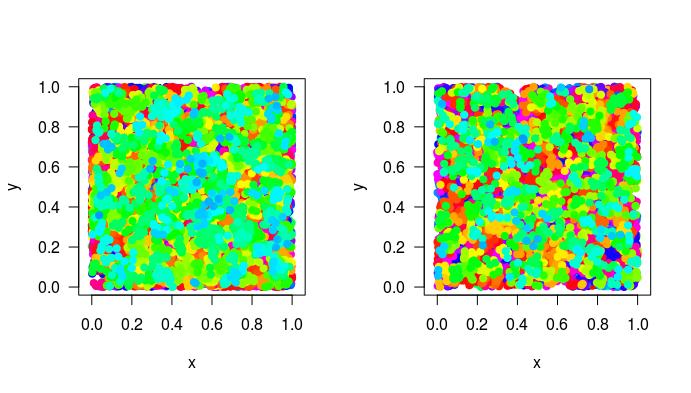Analysis of spatially-explicit community data

The package mobsim offers functions to derive important biodiversity patterns from spatially-explicit community objects, for instance the well-known species- area relationship (SAR). The SAR is generated by nested subsampling of the community with samples of different sizes. To generate the SAR, the function divar (diversity-area relationships) is used, which requires a vector of sampling areas, specified as proportion of the total area. Here, we use an approximately log-scaled sampling area vector ranging from 0.1 - 100% of the total area.

area <- c(0.001,0.002,0.005,0.01,0.02,0.05,0.1,0.2,0.5,1.0)
sar_n_high <- divar(sim_n_high, prop_area = area)
sar_n_low <- divar(sim_n_low, prop_area = area)

For each sampling size, the function calculates mean and standard deviation of several biodiversity indices. For the SAR, we plot the mean species richness vs. sampling area.

names(sar_n_high)
##   "prop_area"      "m_species"      "sd_species"     "m_endemics"
##   "sd_endemics"    "m_shannon"      "sd_shannon"     "m_ens_shannon"
##   "sd_ens_shannon" "m_simpson"      "sd_simpson"     "m_ens_simpson"
##  "sd_ens_simpson"
plot(m_species ~ prop_area, data = sar_n_high, type = "b", log = "xy", ylim = c(2,200),
xlab = "Proportion of area sampled.", ylab = "No. of species",
main = "Species-area relationship")
lines(m_species ~ prop_area, data = sar_n_low, type = "b", col = "red")
legend("bottomright",c("N high","N low"), col = 1:2, pch = 1)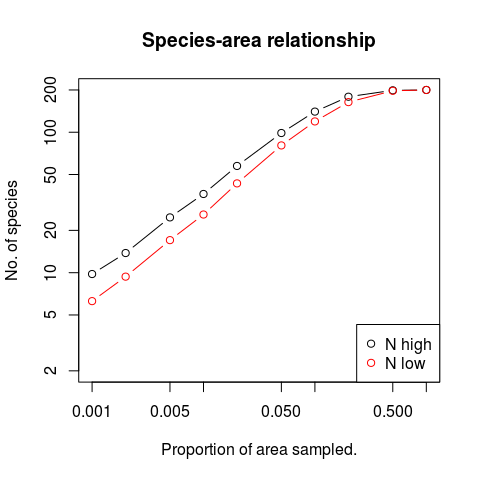We see that there are more species in the landscape with more individuals across most scales. However, the curves converge as soon as 50% or more of the landscape are sampled.

In contrast to the simulated communities, where we have full information about all individuals in the landscape, in reality we only have data from local samples, e.g. plots, traps, etc.. In the following, it is illustrated, how mobsim can be used to simulate the sampling process.

Sampling spatially-explicit community data

The function sample_quadrats distributes sampling quadrats of user defined number (n_quadrats) and size (quadrat_area) in a landscape and returns the abundance of each species in each quadrat. The user can choose different spatial sampling designs. Here, we use a random distribution of the samples.

First, we use many small samples:

par(mfrow = c(1,2))
quadrat_area = 0.001, method = "random",
avoid_overlap = T)
quadrat_area = 0.001, method = "random",
avoid_overlap = T)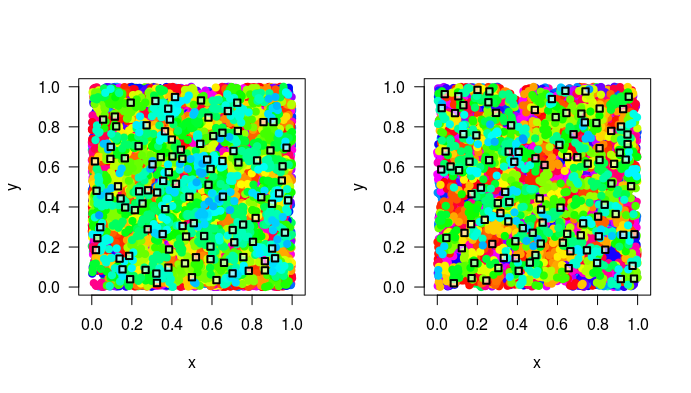Second, we use less, but larger samples, that in total cover the same area as the many small samples used before.

par(mfrow = c(1,2))
quadrat_area = 0.01, method = "random",
avoid_overlap = T)
quadrat_area = 0.01, method = "random",
avoid_overlap = T)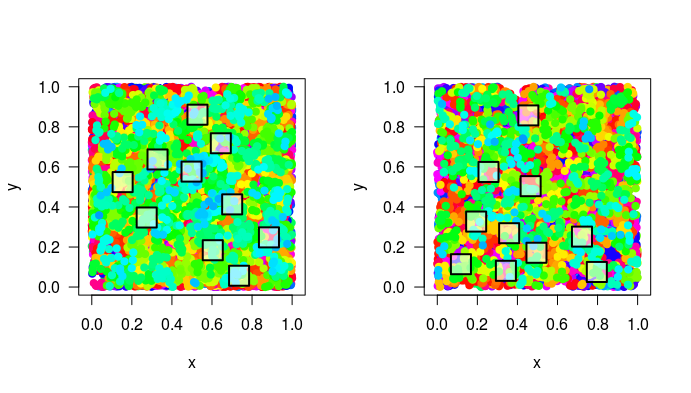The function sample_quadrats return two objects: (i) a community matrix with quadrats in rows, species in columns and the abundance of every species in the respective cell, and (ii) a matrix with the positions of the sampling quadrats in the landscape.

dim(samples_L_n_high$spec_dat) ##  10 200 head(samples_L_n_high$spec_dat)[,1:5]
##       species1 species10 species100 species101 species102
## site1       38         0          0          0          5
## site2       36         0          0          3          0
## site3       62         2          0          0          2
## site4        0         1          0          0          0
## site5        0         9          0          0          0
## site6       82         0          0          0          0
dim(samples_L_n_high$xy_dat) ##  10 2 head(samples_L_n_high$xy_dat)
##               x         y
## site1 0.2240859 0.2971892
## site2 0.5940340 0.6678675
## site3 0.5539087 0.1339117
## site4 0.2781442 0.5868671
## site5 0.6508160 0.3628338
## site6 0.4479799 0.5263771

Analysis of plot-based community data

The community matrix can now be analysed just as ecologist will analyse field data. A software package that is perfectly suited for this and smoothly integrates with mobsim is vegan.

Here, we use vegan to calculate the species richness, the Shannon- and Simpson-diversity indices for both landscapes and for both sampling scales.

Small scale analysis

First, we analyse the small-scale samples:

library(vegan)
S_n_high <- specnumber(samples_S_n_high$spec_dat) S_n_low <- specnumber(samples_S_n_low$spec_dat)

Shannon_n_high <- diversity(samples_S_n_high$spec_dat, index = "shannon") Shannon_n_low <- diversity(samples_S_n_low$spec_dat, index = "shannon")

Simpson_n_high <- diversity(samples_S_n_high$spec_dat, index = "simpson") Simpson_n_low <- diversity(samples_S_n_low$spec_dat, index = "simpson")

The three biodiversity indices are combined into one dataframe and can then be conveniently visualized using boxplots.

div_dat_S <- data.frame(N = rep(c("N high","N low"), each = length(S_n_high)),
S = c(S_n_high, S_n_low),
Shannon = c(Shannon_n_high, Shannon_n_low),
Simpson = c(Simpson_n_high, Simpson_n_low))
par(mfrow = c(1,3))
boxplot(S ~ N, data = div_dat_S, ylab = "Species richness")
boxplot(Shannon ~ N, data = div_dat_S, ylab = "Shannon diversity")
boxplot(Simpson ~ N, data = div_dat_S, ylab = "Simpson diversity")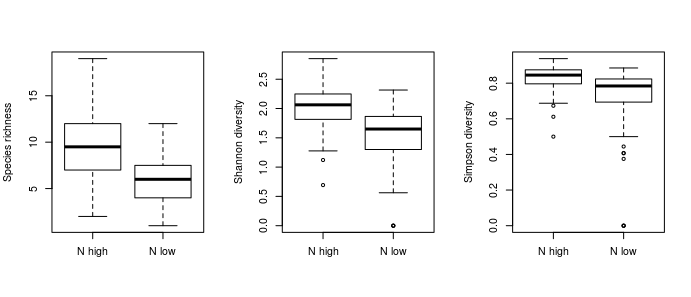We see that on average diversity is reduced for all indices, species richness, Shannon, and Simpson. However, we could like to compare the effects among the three different indices. Since the different diversity indices have different absolute values, we calculate relative changes of the mean values as diversity changes effect size.

The relative change is defined as

$relEff = \frac{diversity(N = low) - diversity(N = high)}{diversity(N = high)}$

Accordingly, a relative effect of -0.5 means that diversity is reduced by 50%, while a positive value means diversity increases by the reduction of total abundance. A value of zero indicates no change.

To calculate the relative change we first average diversity across samples

mean_div_S <- aggregate(div_dat_S[,2:4], by = list(div_dat_S$N), FUN = mean) mean_div_S ## Group.1 S Shannon Simpson ## 1 N high 9.66 2.005570 0.8277624 ## 2 N low 5.88 1.541906 0.7301815 Then we apply the formula shown above to calculate the relative change. relEff_S <- (mean_div_S[mean_div_S$Group.1 == "N low", 2:4] -  mean_div_S[mean_div_S$Group.1 == "N high", 2:4])/ mean_div_S[mean_div_S$Group.1 == "N high", 2:4]
relEff_S
##            S    Shannon    Simpson
## 2 -0.3913043 -0.2311882 -0.1178852

These results indicate that the relative change clearly varies among the indices. While we find a 43% reduction in species richness, there is just a 16% reduction considering the Simpson-index.

Large scale analysis

Now we repeat this analysis using the large samples:

S_n_high <- specnumber(samples_L_n_high$spec_dat) S_n_low <- specnumber(samples_L_n_low$spec_dat)

Shannon_n_high <- diversity(samples_L_n_high$spec_dat, index = "shannon") Shannon_n_low <- diversity(samples_L_n_low$spec_dat, index = "shannon")

Simpson_n_high <- diversity(samples_L_n_high$spec_dat, index = "simpson") Simpson_n_low <- diversity(samples_L_n_low$spec_dat, index = "simpson")
div_dat_L <- data.frame(N = rep(c("N high","N low"), each = length(S_n_high)),
S = c(S_n_high, S_n_low),
Shannon = c(Shannon_n_high, Shannon_n_low),
Simpson = c(Simpson_n_high, Simpson_n_low))
par(mfrow = c(1,3))
boxplot(S ~ N, data = div_dat_L, ylab = "Species richness")
boxplot(Shannon ~ N, data = div_dat_L, ylab = "Shannon diversity")
boxplot(Simpson ~ N, data = div_dat_L, ylab = "Simpson diversity")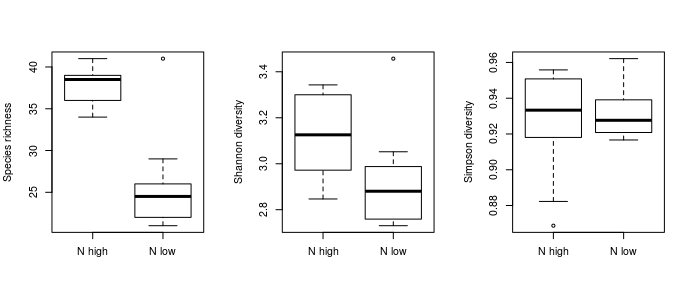mean_div_L <- aggregate(div_dat_L[,2:4], by = list(div_dat_L$N), FUN = mean) relEff_L <- (mean_div_L[mean_div_S$Group.1 == "N low", 2:4] -  mean_div_L[mean_div_L$Group.1 == "N high", 2:4])/ mean_div_L[mean_div_S$Group.1 == "N high", 2:4]

Compare results across scales

Finally, we compare the diversity effect sizes across the two scales.

relEff_S
##            S    Shannon    Simpson
## 2 -0.3913043 -0.2311882 -0.1178852
relEff_L
##            S     Shannon     Simpson
## 2 -0.3129973 -0.06354124 0.004638189

We see that there are differences, both among indices within the same scale, as shown above, but also across scales. The reduction of diversity is smaller at larger scales. However, the change of the effect across scales is weak with species richness, but strong with the Simpson-index.

Conclusion

This simple example hopefully shows two things. First, it illustrates the dependence of biodiversity change on the specific index and the sampling scale used, which even emerges with a very simple change of just reducing the total abundance. Second, it shows the potential of mobsim to investigate and foster understanding of scale- and sampling-dependent biodiversity change. Of course, similar analysis with different changes in biodiversity components, including total abundance, relative abundance and spatial patterns can be easily implemented in mobsim. The simulate changes can then be analysed using different combinations of sampling designs, scales and biodiversity effect sizes.